Dr. J's Maths.com
Where the techniques of Maths
are explained in simple terms.

Calculus - Differentiation - Applied max/min questions.
Type 6: Rates - Miscellaneous questions.

 1. At the entrance to a mine there are electricity wires. The height (H) of the wires in metres above the ground at the mine entrance varies with the outside temperature (t) in degrees Celsius and is given by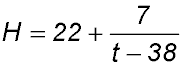When the temperature outside the mine reaches 38° C, all work must stop. (i) Find the height of the wire when the temperature is -1oC. (ii) The company has a special excavator which is 13.3 m high. It is a requirement that all equipment entering the mine must be at least 6.7m below the wires. Find the maximum temperature which will allow the equipment to safely pass under the wire. Answer.(i) H = 21.82 m.(ii) Max temperature is 34.5° C. 2. A light is to be placed over the centre of a circle, radius a units. The intensity I of the light varies as the sine of the angle α at which the rays strike the illuminated surface, divided by the square of the distance d from the light. This relationship can be expressed mathematically as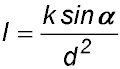. (i) Show that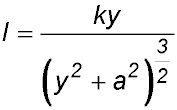. (ii) Find the best height for a light to be placed over the centre of a circle so as to provide the maximum illumination to the circumference. (Do not prove the y value gives you a maximum). Answer.Max height when y = a / √2.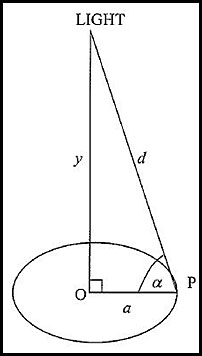3. At a music concert, speakers are placed in two towers (S1 and S2) which are 75 metres apart. The intensity of sound (I) produced by a speaker tower of power P at a distance x metres from the tower is given by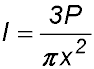. The speakers in tower S1 have a power output of P but the older speakers in tower S2 produce 25% less power.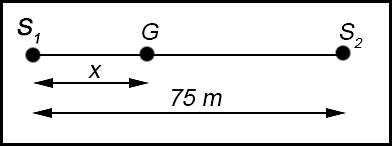The diagram shows Grace stands at G between the two towers and she is x metres from tower S1. (i) Show that the total sound intensity from both speaker towers IT at point G is(ii) Given that the total sound intensity from both speaker towers is IT at point G, find the derivative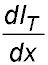. (iii) Hence find the value of x (to the nearest half metre) which minimises the sound intensity so that Grace knows where best to stand so she can enjoy the concert. Answer.Grace stands 39.3 m from S1. .